•Measuring the length and period of a pendulum…

•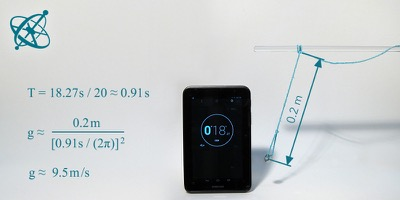…allows you to calculate the local strength of gravity.

•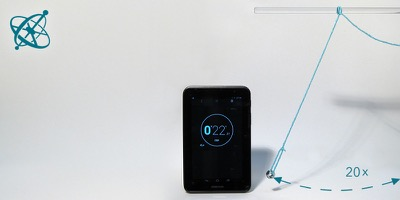With a longer pendulum, though,…

•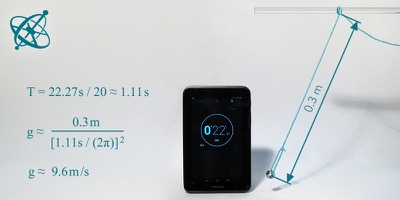…you can improve the accuracy of your measurement.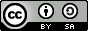www.sciensation.org | Ciênsação hands-on experiments are published as Open Educational resources under a Creative Commons Attribution-ShareAlike 4.0 International License.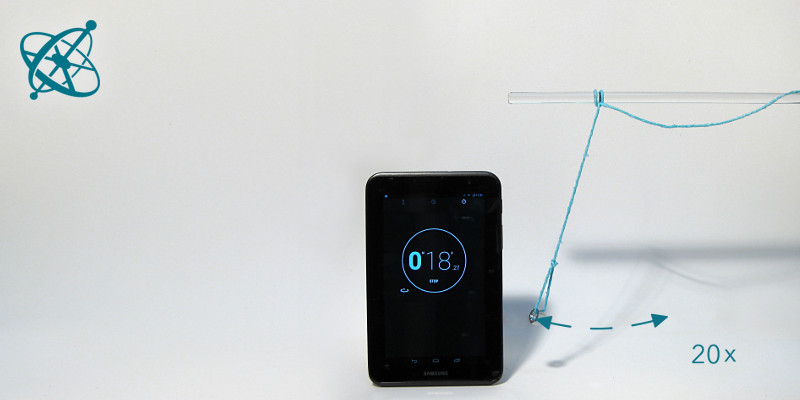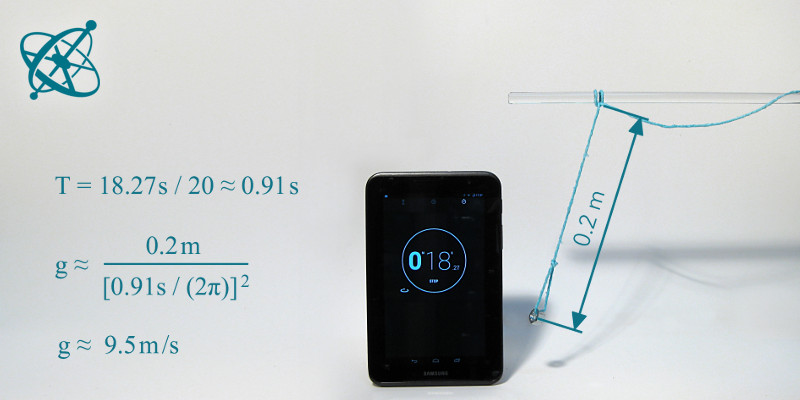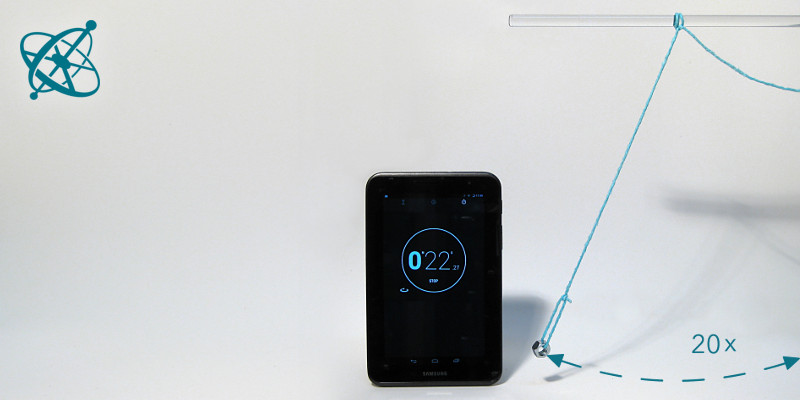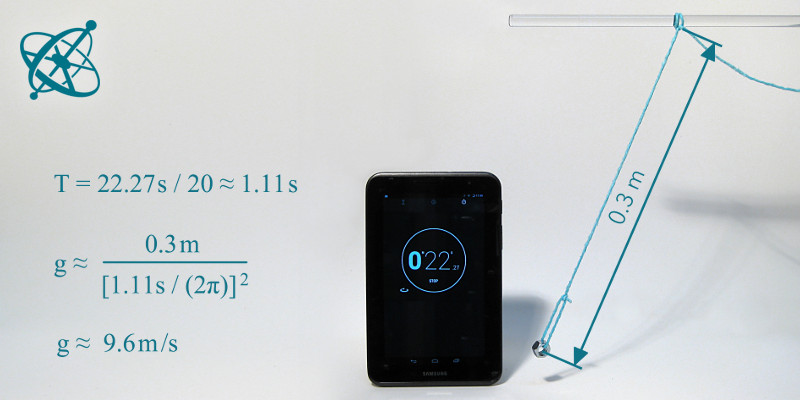Physics Game
age: 14 – 16

# Measuring 'g'

Who takes the most accurate measurement of your local acceleration of gravity g? By turning this into a small competition, your students will be eager to find methods that reduce measurement uncertainties – a valuable skill well beyond this specific experiment.

Learning objective

For small amplitudes, the oscillation period of a pendulum only depends on its length, not its mass.

Taking accurate measurements. Understanding and reducing measurement uncertainties.

String
Weight, e.g. metallic nut
Stop watch (smartphone)

For small amplitudes, the time T it takes a pendulum to complete a swinging cycle is:

T = 2 π sqrt(L/g)

1. Make a simple pendulum from a string and a nut and determine the acceleration of gravity g.

2. How accurate is your measurement?

Guiding Questions (if needed)

Can you solve the equation for g?
g = L/(T/2π)2

What quantities do you need to measure, and how will you do this?
› The length of the pendulum L can be measured with a ruler, the period T by measuring and averaging the time of several swinging periods.

Is T larger for a longer pendulum or a shorter?
› For a longer pendulum.

Is a longer or a shorter pendulum better for an accurate measurement?
› For a longer pendulum both T and L are larger and can be measured with smaller relative error. A longer pendulum also makes it easier to measure at small amplitudes.

Findings

For small amplitudes, the period of a pendulum depends neither on its mass nor amplitude but only on its length. This allows you to calculate the factor g (which depends on your local strength of gravity) just by measuring the period and length of the pendulum.

Discuss with your students how accurate they can measure each of these quantities, and why a longer pendulum, and averaging over more swinging periods, helps to reduce the total measurement uncertainty.